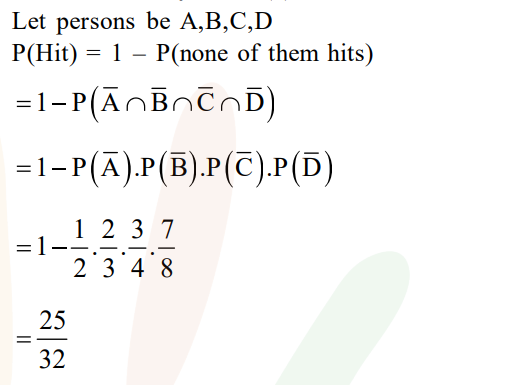# Four persons can hit a target correctly with`
Question:

Four persons can hit a target correctly with

probabilities $\frac{1}{2}, \frac{1}{3}, \frac{1}{4}$ and $\frac{1}{8}$ respectively. if all

hit at the target independently, then the probability that the target would be hit, is

1. $\frac{25}{192}$

2. $\frac{1}{192}$

3. $\frac{25}{32}$

4. $\frac{7}{32}$

Correct Option: , 3

Solution: